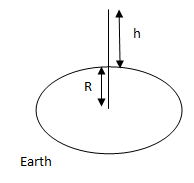Courses
Courses for Kids
Free study material
Offline Centres
MoreLast updated date: 27th Nov 2023
Total views: 278.4k
Views today: 3.78k

# The escape velocity of a body from the earth’s surface is ${V_e}$. The escape velocity of the same body from a height equal to $7R$ from the earth’s surface will beVerified
278.4k+ views
Hint: The equation for the speed is often derived by applying the Law of Conservation of Energy. Once an object—such as a rocket—has reached a sufficient velocity above the surface of a moon, planet, or sun. And it is not any longer being powered, it's an initial gravitational P.E. and an initial K.E.

Formula used: A satellite orbits at a height h above the Earth's surface. Assume R be the Earth's radius,${V_{{e_1}}}$ be the escape velocity and ${V_{{e_2}}}$ be the orbital velocity of the satellite orbiting at a height which is equal to $7R$ , then we need to find them ${V_{{e_2}}}$ at this height -
We have a relationship that is, the relation between escape velocity and earth’s radius –
${V_e} = \sqrt {\dfrac{{2GM}}{R}}$

Complete step-by-step solution:
Earth’s escape velocity = ${V_{{e_1}}} = \sqrt {\dfrac{{2GM}}{R}}$…........(1)
Escape velocity from height h = ${V_{{e_2}}} = \sqrt {\dfrac{{2GM}}{{R + h}}}$
$= \sqrt {\dfrac{{2GM}}{{R + 7R}}}$ (as $h = 7R\;$)…........(2)From (1) and (2), rearranging the equations - $\dfrac{{{V_{e2}}}}{{{V_{e1}}}} = \sqrt {\dfrac{1}{8}}$
${V_{e2}} = \dfrac{{Ve}}{{\sqrt {1/8} }}$ (Since $Ve1 = Ve$)
${V_{e2}} = \dfrac{1}{{2\sqrt 2 }} \times Ve$
Thus, the Escape velocity for the body projected from height 7R from the earth surface is $\dfrac{1}{{2\sqrt 2 }}$ times of Earth escape velocity.

Note: The escape velocity from Earth’s surface is about $11,186{\text{ }}m/s$ The escape velocity is not dependent on the mass of the body and the direction of projection. It depends on the gravitational potential at the point from the place the body is projected.
The potential energy at infinity is taken as 0. The total minimum energy at infinity in case of the escape velocity should be zero. If an object is thrown with a velocity less than escape velocity, then it will have energy less than zero and will be negative.
There exists a relationship between speed and orbital velocity. The connection between speed and orbital velocity is proportional in nature. Speed refers to the minimum velocity needed to beat the gravitational pull of the huge to fly to the initiated space. Orbital velocity may be a velocity that's required to rotate around a huge body.